Lévy metric

(diff) ← Older revision | Latest revision (diff) | Newer revision → (diff)

A metricin the spaceof distribution functions (cf. Distribution function) of one-dimensional random variables such that: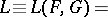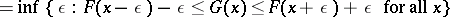for any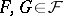. It was introduced by P. Lévy (see ). If between the graphs ofandone inscribes squares with sides parallel to the coordinate axes (at points of discontinuity of a graph vertical segments are added), then a side of the largest of them is equal to.

The Lévy metric can be regarded as a special case of the Lévy–Prokhorov metric. The definition of the Lévy metric carries over to the setof all non-decreasing functions on(infinite values of the metric being allowed).

Most important properties of the Lévy metric.

1) The Lévy metric induces a weak topology in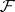(cf. Distributions, convergence of). The metric space () is separable and complete. Convergence of a sequence of functions fromin the metricis equivalent to complete convergence.

2) If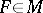and if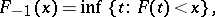then for any,3) Regularity of the Lévy metric: For any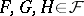,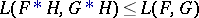(heredenotes convolution, cf. Convolution of functions). A consequence of this property is the property of semi-additivity: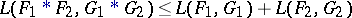and the "smoothing inequality" :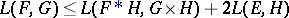(being a distribution that is degenerate at zero).

4) If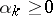,, then5) If,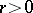, is an absolute moment of the distribution, then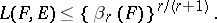6) The Lévy metric onis related to the integral mean metric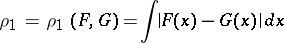by the inequality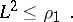7) The Lévy metric onis related to the uniform metricby the relations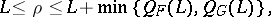(*)

where(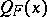is the concentration function for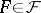). In particular, if one of the functions, for example, has a uniformly bounded derivative, then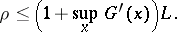A consequence of (*) is the Pólya–Glivenko theorem on the equivalence of weak and uniform convergence in the case when the limit distribution is continuous.

8) If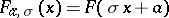, whereandare constants, then for any,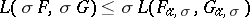(in particular, the Lévy metric is invariant with respect to a shift of the distributions) and9) Ifandare the characteristic functions (cf. Characteristic function) corresponding to the distributionsand, then for any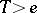,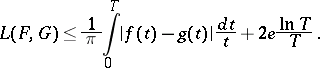The concept of the Lévy metric can be extended to the case of distributions in.